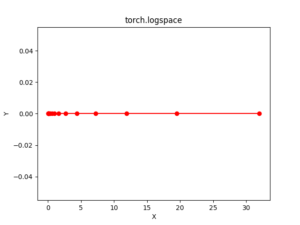# Python Pytorch logspace() method

PyTorch is an open-source machine learning library developed by Facebook. It is used for deep neural network and natural language processing purposes.

The function torch.logspace() returns a one-dimensional tensor of steps points logarithmically spaced with base base between.

The output tensor is 1-D of size steps.

Syntax: torch.logspace(start, end, steps=100, base=10, out=None)

Parameters:
start: the starting value for the set of point.
end: the ending value for the set of points
steps: number of points to sample between start and end. Default: 100.
base: base of the logarithm function. Default: 10.0
out(Tensor, optional): the output tensor

Return type: A tensor

Code #1:

 # Importing the PyTorch library  import torch     # Applying the logspace function and  # storing the resulting tensor in 't'  a = torch.logspace(3, 10, 5)  print("a = ", a)     b = torch.logspace(start =-10, end = 10, steps = 5)  print("b = ", b)

Output:

a =  tensor([1.0000e+03, 5.6234e+04, 3.1623e+06, 1.7783e+08, 1.0000e+10])
b =  tensor([1.0000e-10, 1.0000e-05, 1.0000e+00, 1.0000e+05, 1.0000e+10])


Code #2: Visualization

 # Importing the PyTorch library  import torch  # Importing the NumPy library  import numpy as np     # Importing the matplotlib.pylot function  import matplotlib.pyplot as plt     # Applying the logspace function to get a tensor of size 15 with values from -5 to 5 using base 2  a = torch.logspace(-5, 5, 15, 2)  print(a)     # Plotting  plt.plot(a.numpy(), np.zeros(a.numpy().shape), color = 'red', marker = "o")   plt.title("torch.linspace")   plt.xlabel("X")   plt.ylabel("Y")      plt.show()

Output:

tensor([3.1250e-02, 5.1271e-02, 8.4119e-02, 1.3801e-01, 2.2643e-01, 3.7150e-01,
6.0951e-01, 1.0000e+00, 1.6407e+00, 2.6918e+00, 4.4164e+00, 7.2458e+00,
1.1888e+01, 1.9504e+01, 3.2000e+01])
[torch.FloatTensor of size 15]My Personal Notes arrow_drop_upCheck out this Author's contributed articles.

If you like GeeksforGeeks and would like to contribute, you can also write an article using contribute.geeksforgeeks.org or mail your article to contribute@geeksforgeeks.org. See your article appearing on the GeeksforGeeks main page and help other Geeks.

Please Improve this article if you find anything incorrect by clicking on the "Improve Article" button below.

Article Tags :

Be the First to upvote.

Please write to us at contribute@geeksforgeeks.org to report any issue with the above content.